# Computing vibration–rotation-tunnelling levels of HOD dimer

Xiao-Gang Wang and Tucker Carrington JrChemistry Department, Queen's University, Kingston, Ontario K7L 3N6, Canada. E-mail: xgwang.dalian@gmail.com; Tucker.Carrington@queensu.ca; Fax: +1-613-533-6669

Received 13th July 2018 , Accepted 10th August 2018

First published on 11th August 2018

Using an accurate 6D water dimer potential energy surface, we compute vibration–rotation-tunnelling levels of HOD dimer, by assuming that the two monomers are rigid. HOD dimer has two isomers, a D-bonded isomer and an H-bonded isomer, and the wavefunctions of both isomers have amplitude in four wells. HOD dimer is important because, unlike the case of H2O dimer or D2O dimer, it is possible to measure the largest tunnelling splitting. Results for HOD dimer, therefore facilitate the testing of H2O dimer potentials. In J. Chem. Phys., 1995, 102, 1114, experimental results were interpreted in terms of 1D models. Experimental splittings of both isomers, obtained by fitting an energy level equation to spectra, are in good agreement with those we compute.

## I. Introduction

Water dimer has been studied for decades by both experimentalists1–12 and theorists.13–28 It is hoped that understanding the structure of the water dimer energy levels and determining an accurate water dimer potential energy surface (PES) will aid in modelling the effect of hydrogen bonds in biological molecules and chemical reactions in condensed phases. Good models for bulk water are built on the potential energy surface of water dimer. The water dimer itself is thought to play an important role in Earth's atmosphere. The water dimer PES is far from harmonic. It has 8 accessible (by feasible permutation-inversion operations) identical wells separated by low barriers. As a consequence, tunnelling is important and the dynamics is complicated. Although it is possible to identify paths between the minimum (and barrier heights along these paths provide valuable information), it is very difficult to develop useful low-dimensional models. For example, the usefulness of 1D models is limited by coupling between vibrational coordinates and by the difficulty of choosing the mass to use in the 1D kinetic energy operator. In this paper, we present results of calculations on HOD dimer done by assuming that the monomers are rigid, but including all six of the inter-monomer coordinates. Rigid monomer calculations are quite accurate, when the monomers are not excited, for HF dimer29 and water dimer.16–19,28

The isotopologue obtained by replacing two protons with deuterons has four isomers. The two that can be described as HOD dimer are shown in Fig. 1. The two that can be described as H2O–D2O are shown in Fig. 2. No feasible permutation-inversion operations convert the isomers in Fig. 1(2) to isomers in Fig. 2(1). The isotopologue (H2O–HDO) obtained by replacing one proton with a deuteron has three isomers, shown in Fig. 3. The isotopologue (D2O–HDO) obtained by replacing three protons with deuterons has three isomers, shown in Fig. 4. In this paper, we study HOD dimer (Fig. 1) which has an HDO–DOH (D-bonded isomer) and an HDO–HOD (H-bonded isomer). The atom immediately after the hyphen indicates the H/D atom forming the hydrogen bond with the O atom.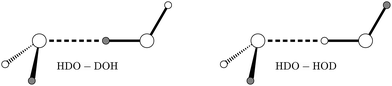Fig. 1 Two isomers of HOD dimer: D-bonded (HDO–DOH) isomer on the left, H-bonded (HDO–HOD) isomer on the right.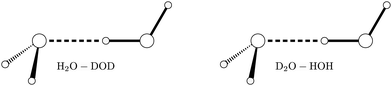Fig. 2 Two isomers of H2O–D2O.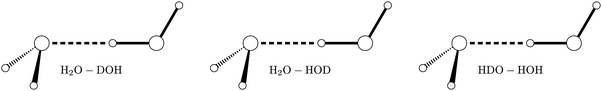Fig. 3 Three isomers of H2O–HDO.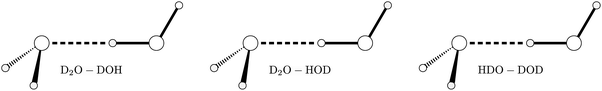Fig. 4 Three isomers of D2O–HDO.

In H2O dimer, tunnelling among the 8 wells splits each ro-vibrational level into six levels. The six levels consist of two triple forks. The upper (lower) prong of each triple fork is a B(A) or an A(B) level. The middle prong is an E state. The spectrum is therefore characterised by three splittings.16,17 The largest splitting is the difference between the average energy of the upper and lower prongs of the top fork and the average energy of the upper and lower prongs of the bottom fork. This largest splitting is called the acceptor switch (AS) splitting. The second largest splitting is the difference between the top and bottom prongs of a fork. This splitting is called the interchange splitting. The third type of splitting, often called the bifurcation splitting is very small. Tunnelling along the AS path does not break the hydrogen bond and the AS path therefore has the lowest barrier.

Due to its importance, there are several good PESs for (H2O)2. According to the Born–Oppenheimer approximation, a PES for (H2O)2 should also be valid for (HOD)2. To verify the accuracy of a PES, it is always a good idea to confirm that spectra computed for all isotopologues agree well with experimental data. For (H2O)2 this is particularly important because the AS splitting cannot be measured. Transitions between the A (or B) level in the bottom fork and the A (or B) level in the top fork are forbidden by symmetry. Although transitions from the E level of the bottom fork to the E level of the top fork are in principle possible, they have never been measured due to their weak intensities. See however ref. 9. Only the sum of the AS tunnelling splitting for K = 0 and K = 1 has been measured. K is the quantum number for the projection of the total angular momentum on the inter-monomer axis, −JKJ.

Each of the two HOD dimer isomers has four accessible wells. Each ro–vibrational level is therefore split into four states. HOD dimer has only two types of tunnelling splittings: the AS and interchange splittings. There is no bifurcation splitting because the H and D nuclei have different masses. As is the case for (H2O)2, the larger (AS) tunnelling splitting arises from a motion which interchanges the H and D on the acceptor monomer. This tunnelling is called methyl-amine-type internal-rotation motion in ref. 30 and 31. The smaller tunnelling splitting arises from the interchange of the donor and acceptor roles of the two monomers. In HOD dimer it is possible to measure the AS splitting for a single value of K. This makes it possible to compare theoretical AS tunnelling splittings with experimental values, thereby testing the accuracy of the PES. Experimental values for K = 0 and K = 1 are known for both isomers of HOD dimer.30

Since no transitions between levels of different isomers were found, there is nothing known experimentally about their relative energies. Our calculation shows that the ground state of D-bonded isomer is lower than that of the H-bonded isomer by 57.37 cm−1.

## II. Evaluating the PES in HOD dimer coordinates

According to the Born–Oppenheimer approximation, one can calculate energy levels of HOD dimer using an (H2O)2 PES. To do this, we must evaluate the (H2O)2 PES at values of the internal coordinates used for HOD dimer. We compute the spectra of HOD dimer assuming the monomers are rigid. The coordinates we use are denoted (α, β; ΩA; ΩB; r0).32 See Fig. 5. The orientation of the molecule-fixed (MF) frame of monomer X with respect to the dimer-fixed (DF) frame attached to the vector from the centre of mass of monomer A to the centre of mass of monomer B,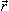0, is specified by the Euler angles ΩX, X = A, B. r0 is the length of0, the vector from the centre of mass of monomer A to the centre of mass of monomer B. α and β specify the orientation of0 with respect to a space-fixed (SF) frame. The axes of the MF frames are the principal axes of the monomers. We use the 6D water dimer PES called CCpol-8s19 in the literature. Its input is the internal coordinates of (H2O)2, denoted in this paper (ΩA′; ΩB′; r0′). When (H2O)2 and (HOD)2 have the same shape they have the same potential value, however, (ΩA; ΩB; r0) and (ΩA′; ΩB′; r0′) are different, because the coordinates are mass dependent. One must therefore convert (ΩA; ΩB; r0) to (ΩA′; ΩB′; r0′) so that the potential can be evaluated.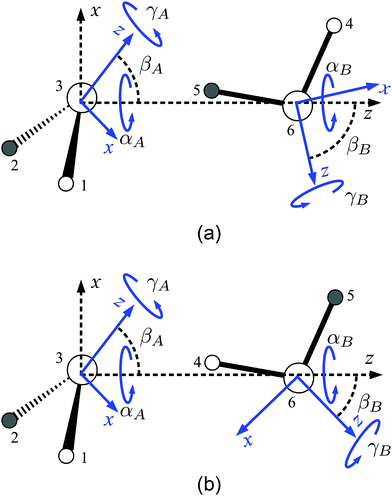Fig. 5 The angles defining the MF and DF frames for the D-bonded (HDO–DOH) isomer, panel (a), and the H-bonded (HDO–HOD) isomer, panel (b) of HOD dimer. The DF frame is indicated by dashed x–z axes. The two MF frames are indicated by blue x–z axes. The A MF frame is the principal axes frame with the z-axis along the b-axis and such that the z coordinate of atom 3 is positive, and the x-axis in the HDO plane and such that the x coordinate of atom 1 of monomer is positive. The D-atom is black. With the acceptor at the left, labelled as A, and donor on the right, labelled as B, the equilibrium coordinates are: (αA, βA, γA; αB, βB, γB) = (0.0°, 58.3°, 104.7°; 205.5°, 82.1°, 179.2°); r0e = 5.4419a0, for the D-bonded isomer; and (αA, βA, γA; αB, βB, γB) = (0.0°, 57.6°, 105.1°; 206.5°, 40.9°, −1.2°); r0e = 5.5771a0, for the H-bonded isomer.

To explain how this is done we refer to (HOD)2 as the minor isotopologue and (H2O)2 as the major isotopologue. We first (i) convert (ΩA; ΩB; r0) to Cartesian coordinates in the DF frame of the minor isotopologue and then (ii) convert these Cartesian coordinates to (ΩA′; ΩB′; r0′). Step (ii) would not be required if the input to the PES were Cartesian coordinates (as is sometimes the case, e.g. in the PESs HBB2,33 WHBB,26 MB-pol27).

The DF frame Cartesian coordinates (step (i)), of atom i in monomer A, ξDFAi are obtained from the MF frame Cartesian coordinates by a rotation

 ξDFAi = St(αA, βA, γA)ξMFAi
 ξDFBi = St(αB, βB, γB)ξMFBi + (0, 0, r0)t, (1)
where S is the direction cosine matrix defined in ref. 34. ξMFAi are the Cartesian coordinates of atom i of minor isotopologue monomer in the MF frame. They are easily calculated once the frame is known. The MF frames are principal-axis frames for the rigid monomers. Note that the orientation of the minor MF frame is tilted with respect to the orientation of the bisector frame (which is the same as the principal axes frame for H2O). See Fig. 6. Eqn (1) could also be used if the monomers were not rigid.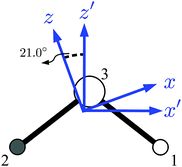Fig. 6 The MF frame (x−z) for HOD and the MF frame (x′−z′) for H2O. The HDO MF frame is tilted with respect to the H2O MF frame by 21.0°. The origin of the H2O MF frame is shifted with respect to the origin of HDO MF frame. Atom 1 is H and atom 2 is D.

Knowing the DF frame minor Cartesian coordinates for a particular shape, we wish to find the corresponding major internal coordinates (step (ii)). To do this we first determine the orientation of the major MF frames. The major MF frames are bisector frames. For example, for monomer A, the unit vectors are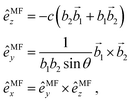(2)
where,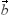i = ξDFiξDF3 is a bond vector for OHi for monomer A and c is a positive normalization constant. θ is the angle between1 and2. Even though these unit vectors are for the major isotopologue, there is no need to attach a prime superscript since they are clearly defined in eqn (2). We also need,0′, the vector pointing from the centre of mass of monomer A to that of monomer B. From the MF frame unit vectors and vector0′, one can determine the last two Euler angles for both monomers (A and B labels are not indicated),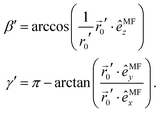(3)
These equations are obtained by recognizing that the spherical polar angles of0′ in the MF frame are (β′, π−γ′). They are equivalent to (−β′, −γ′), but we use (β′, π−γ′) because the polar angle cannot be negative. It remains to find αB′ − αA′. This is done via intermediate (one for each monomer) frames, labelled by “int”, which are obtained by rotating the dimer-fixed frame about its z-axis by α′. Both the intermediate frames have the same z axis. Unit vectors for the intermediate frames can be obtained from the unit vectors of the MF frames (eqn (2)), by recognizing that by rotating an intermediate frame about its y-axis by β′ and then about its z-axis by γ′ where β′ and γ′ are given in eqn (3) one obtains a MF frame. Therefore, the x unit vector of an intermediate frame is
 êintx = S1,1(0, β′, γ′)êMFx + S2,1(0, β′, γ′)êMFy + S3,1(0, β′, γ′)êMFz. (4)
The dihedral angle between the plane that contains the z axis and the x axis of the A intermediate frame and the plane that contains the z axis and the x axis of the B intermediate frame is αB′ − αA′.

Some water dimer PESs (such as SAPT-5st17) depend on Cartesian coordinates of sites attached to the monomers. For example, in the SAPT-5st case, from the H2O dimer PES code, it is straightforward to make a code for the D2O dimer PES by recomputing the position of the 5 sites. This is done by shifting the origin of the Cartesian axes from the centre of mass of D2O to the centre of mass of H2O (a simple shift along the C2 axis). In fact the authors of the SAPT-5st PES provide H2O dimer and D2O dimer options. We use their D2O dimer option to test our coordinate transformation. Values of their D2O dimer PES and values we obtain by transforming the internal coordinates of D2O–D2O to the internal coordinates of H2O–H2O agree. We also compute ro-vibrational energy levels with both approaches and they agree as well.

## III. Calculation details

We use the kinetic energy operator of Brocks et al.32 and the CCpol-8s19 PES. Eigenvalues and eigenvectors of a basis representation of the Hamiltonian operator are computed with the Lanczos algorithm. Quadrature is used for potential matrix elements and matrix-vector products are evaluated by doing sums sequentially.35–38 To do the calculation we must know (for the kinetic energy operator) the rotational constants of HOD and (to evaluate the PES) the orientation of the MF frames. The rotational constants for HDO are taken to be the experimental values,39A = 23.41911, B = 6.40655, and C = 9.10311 cm−1. The MF frames are principal axis frames. To find the orientation of the principal axes we need the tilt angle referred to in Section II. To get the tilt angle, it would be ideal to use the vibrational ground state averaged geometry of HOD. This, however, is inconsistent with the 6D PES which is constructed assuming the geometry of the monomers is the ground state averaged geometry of H2O. To determine the tilt angle, we therefore use the vibrational ground state averaged geometry of H2O, but when computing the moments and products of inertia we replace one of the H with D. The H2O ground state averaged geometry we use is r(O–H) = 1.8361063 Bohr, θ(H–O–H) = 104.69°.17 The tilt angle is 21.0°. See Fig. 6.

### A. Basis

We choose to use the most accurate 6D (H2O)2 PES, CCpol-8s.19 6D (H2O)2 vibration–rotation-tunnelling (VRT) levels have been computed previously.19,24,28 The 6D HOD dimer calculations are done with the parity-adapted ro-vibrational basis functions of ref. 28. They are linear combinations of products of three symmetric-top eigenfunctions. The monomer symmetric-top eigenfunctions are labelled by jX, mX, kX, X = A, B. For the calculations of this paper, the maximum value of jX, mX, kX is 14. Nr0 = 9 is the number of PODVR (potential optimised discrete variable representation) points. The numbers of angular quadrature points are Nθ = 15 (for βA, βB) and Nϕ = 30 (for αAαB, γA, γB). The same PODVR basis40,41 was used in the 6D calculation of H2O dimer in ref. 28 where it was shown that levels up to 150 cm−1 are converged to within 0.001 cm−1. The PODVR functions are calculated using a basis of 200 sine functions in a box from 4.2 to 10.0 Bohr for r0. We use the G4 permutation–inversion group in the calculation. The permutation–inversion group consists of 4 symmetry operations: {E, E*} ⊗ {E, Eab} where E* is the inversion operation and Pab permutes the two monomers. G4 has four symmetry labels: A+, A, B+, and B. +/− label even/odd symmetry under E* and A/B label symmetric/antisymmetric irreps under Pab.

To define the PODVR basis, we need to know the equilibrium structure. It was determined by finding the values of the HOD dimer coordinates that minimize the potential (using the coordinate transformation of Section II). The minima for the D-bonded and H-bonded isomers have the same energy. The r0 reference potential used to define the PODVR basis is the cut potential for which the other coordinates have their values at the D-bonded isomer minimum (the cut potential for which the other coordinates have their values at the H-bonded isomer minimum should be very similar). The equilibrium structures of the D-bonded and H-bonded isomers of HOD dimer on the CCpol-8s PES are given in Table 1. The minimum energy is −1785.16 cm−1, the same as the minimum energy of H2O dimer. Compared with the equilibrium structure of the H2O on the same PES: (αA, βA, γA; αB, βB, γB) = (0.0°, 55.90°, 90.0°; 180°, 60.55°, 0°), and r0e = 5.5045a0, the biggest difference is in βB (the angle associated with the donor). βB increases by roughly 20° for the D-bonded isomer and decreases by roughly 20° for the H-bonded isomer. This change is due largely to the z principal axis of the HDO monomer being tilted by 21.0° from the z principal axis of the H2O monomer, shown in Fig. 6. The D-bonded isomer has a smaller r0e simply because the centre of mass of the donor is closer to the acceptor than in the H-bonded isomer. The biggest difference between the coordinates of the H-bonded and D-bonded isomers is the γB value. The D-bonded value is about π larger than its H-bonded counterpart, i.e., the donor, on the right, is rotated by π about the z-axis of its MF frame.

Table 1 The minium structure of the D-bonded (HDO–DOH) and the H-bonded (HDO–HOD) isomer of HOD dimer in the dynamical coordinates on the CCpol-8s potential energy surface, with the acceptor on the left and the dimer-fixed frame attached as in Fig. 5. See also Fig. 1
HDO–DOH (D-bonded) HDO–HOD (H-bonded)
(αAe, βAe, γAe) (deg) (0, 58.3, 104.7) (0, 57.6, 105.1)
(αBe, βBe, γBe) (deg) (205.5, 82.1, 179.2) (206.5, 40.9, −1.2)
r 0e (Bohr) 5.4419 5.5771

Table 2 J = 0 energy levels of the D-bonded (HDO–DOH, labelled by D) and the H-bonded (HDO–HOD, labelled by H) isomer of HOD dimer (in cm−1). “m” means that the state is a mixture of the D-bonded and H-bonded isomers. The second label after the energy is K. All the levels up to 200 cm−1 are given. All levels are relative to the ZPE of D-bonded isomer −1199.2443 cm−1. The ZPE of the H-bonded isomer is 57.3679 cm−1 higher, indicated in bold face
J = 0, A+ J = 0, B+ J = 0, A J = 0, B
1 0.0000 D 0 0.0484 D 0 7.9243 D 0 7.8887 D 0
2 57.3679 H 0 57.5360 H 0 62.0371 H 0 61.8835 H 0
3 59.3109 D 0 59.6806 D 0 89.3466 D 0 88.6759 D 0
4 96.8388 D 0 97.0955 D 0 101.9852 D 0 101.9955 D 0
5 104.5395 D 0 106.0817 D 0 120.3539 D 0 120.2938 D 0
6 111.5243 H 0 111.8889 H 0 138.1983 H 0 138.0850 H 0
7 118.0563 D 0 119.7084 D 0 148.7331 D 0 147.6840 D 0
8 146.8093 D 0 146.9133 D 0 156.2844 H 0 154.7724 H 0
9 149.2467 H 0 149.1057 H 0 157.8954 D 0 156.5962 D 0
10 153.4771 H 0 154.6986 H 0 159.2012 H 0 159.0841 H 0
11 154.4936 D 0 162.7574 m 0 169.8538 D 0 166.9860 D 0
12 159.5300 H 0 164.5240 m 0 185.9490 D 0 183.7684 D 0
13 169.5734 D 0 170.9740 D 0 193.2346 D 0 194.5436 D 0
14 183.0715 D 0 187.1242 D 0 196.4665 H 0 197.0753 m 0
15 186.6550 D 0 188.6736 D 0 199.3191 H 0
16 194.8086 D 0 196.9386 m 0
17 197.2810 m 0 0

### B. Distinguishing D-bonded and H-bonded isomers

The D-bonded and H-bonded isomers of HOD dimer correspond to two different sets of four wells on the same PES. We calculate solutions of the Schroedinger equation on the PES and therefore obtain both wavefunctions localized in the D-bonded wells and in the H-bonded wells by solving one matrix eigenvalue problem. To label states as being D-bonded or H-bonded, we examine wavefunctions. States localized in the D-bonded and H-bonded wells have different coordinate ranges. The appropriate coordinate ranges are apparent from the equilibrium structures of the two isomers (Table 1). The largest difference between the two structures is the value of the last Euler angle of the donor: γD (or γB if we put the donor on the right and label it as B). The D-bonded isomer has γB values near 180°. The H-bonded isomer has γB values near 0°. By rotating the donor by 180° about its C2 axis, one moves to the H-bonded well from D-bonded well. The two isomers also have somewhat different values of βB: 82° and 41°. This is due to the tilt of the HOD MF frame. The difference between them is about twice the value of the tilt angle. The simplest way to distinguish the isomers is to fix all other coordinates and examine wavefunctions as a function of γB. The exact values used for the other coordinates is not important; we use averages for the equilibrium geometries of the two isomers: βA = 58°, γA = 105°, βB = 61°, and r0 = 5.44 Bohr. In Fig. 7, we plot 2D cuts ΨαAαB,γB of selected states to demonstrate how we identify the two isomers. For the rotational coordinates, arbitrary values α = β = 45° are chosen. Once we have sorted the levels into two groups, one for H-bonded and one for D-bonded, we find that the energy levels of both isomers have the same symmetry pattern.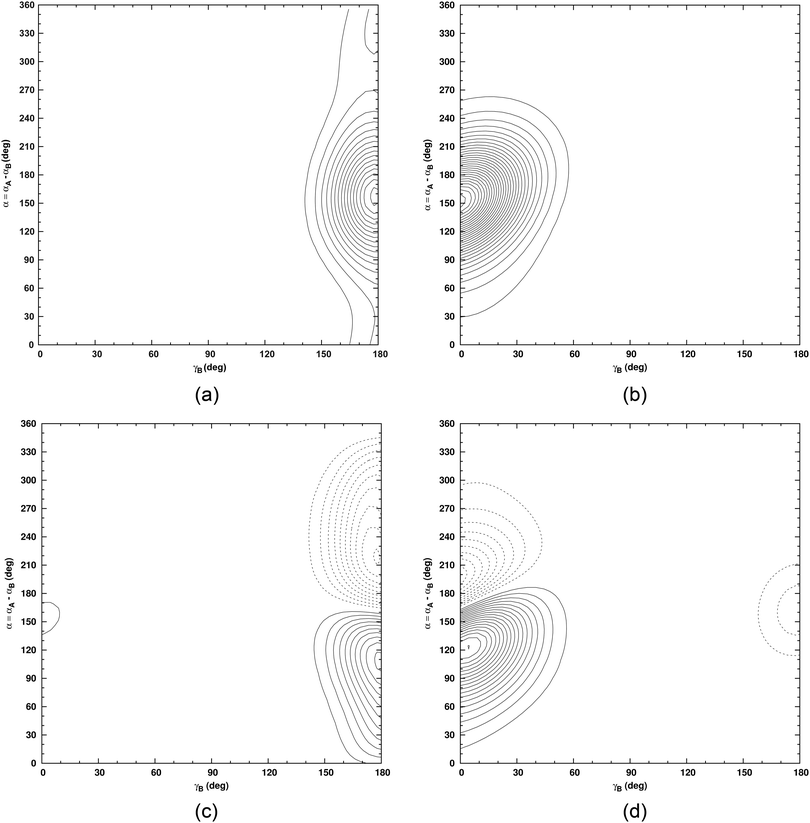Fig. 7 Wavefunction cuts for the D-bonded isomer: ground state at 0 cm−1 (A+) (a) and first excited state at 59.31 cm−1 (A+) (c). Wavefunction cuts for the H-bonded isomer: ground state at 57.37 cm−1 (A+) (b) and first excited state at 111.52 cm−1 (A+) (d).

## IV. Results and discussion

The ground state energy level structures of the D-bonded and H-bonded isomers are the same and shown in Fig. 8 and 9, respectively. Each rotational level is split into four levels by tunnelling. Following Fig. 1 of Karyakin et al.,30 the four sub-levels for K = 0 are labelled as V = 0, 1, 2, and 3, and the four sub-levels for |K| = 1 are labelled as V = 4, 5, 6, and 7. V labels a group of levels with different J and the same |K|. Each level is labelled by (V, J, K). Following Karyakin et al., when |K| > 0 and two levels with the same |K| are nearly degenerate, the lower of the two is labelled with K = −|K|. We assign |K| to each of our levels by analysing the calculated wavefunctions.42 In their figure, Karyakin et al.30 show only J = 1 levels. Fig. 8 includes also J = 0 levels. This makes it easier to visualize transitions. The symmetry labels of Fig. 8 (obtained from the symmetry-adapted Lanczos method43,44) match perfectly those of Fig. 4 of the effective tunnelling Hamiltonian model (internal-axis-method, IAM) of Coudert and Hougen.45 The dipole transition selection rule is A+ ↔ A and B+ ↔ B.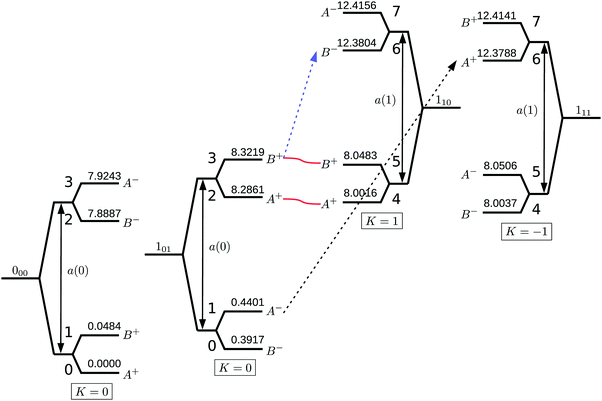Fig. 8 Schematic diagram of J = 0 and J = 1 levels of the D-bonded isomer of HOD dimer.45 Each level is labelled by a value of V (see the text). Two observed transitions are indicated: a b-type transition (in blue) between V = 3 and 6 at 104.828 GHz, and a c-type transition (in black) between V = 1 and 6 at 222.054 GHz for H-bonded isomer. The difference of these two transitions could be used to determine a(0). Values of the calculated J = 0 and J = 1 levels of the D-bonded isomer of HOD dimer are also indicated.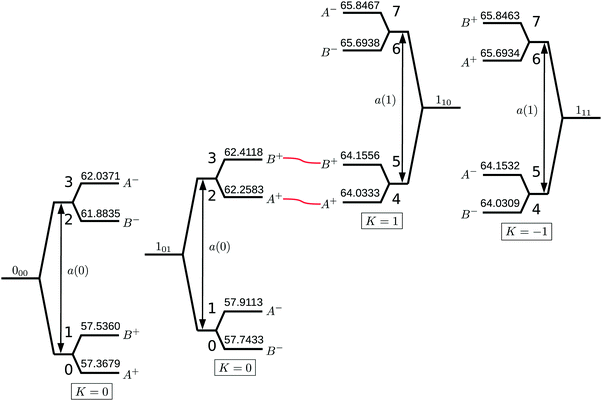Fig. 9 All calculated J = 0 and J = 1 levels of H-bonded isomer of HOD dimer.

For HOD dimer Karyakin et al. determine splittings by choosing the parameters of the simple energy level expression

 E(J, K, V) = EV +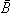V[J(J + 1) − K2] − DV[J(J + 1) − K2]2 + ((B − C)V/4)KJ(J + 1) (5)
so that differences between different E(J, K, V) reproduce their experimental transition frequencies. EV is the origin for the group of levels labelled by V. Note that K may be positive or negative. Two nearly degenerate levels labelled by +K and −K have the same EV.

For H2O dimer and D2O dimer, only a- and c-type transitions are observed. The b-type transitions are absent because the component of the dipole perpendicular to the Cs symmetry plane is zero. The absence of b-type transitions prevents the determination of AS tunnelling splittings, denoted a(K). For both the D-bonded and the H-bonded isomers of HOD dimer, a-, b- and c-type transitions between V levels are observed.30 For each J, there are four symmetry-allowed b-type and four symmetry-allowed c-type transitions between K = 0 and |K| = 1 levels. All of them are observed by Karyakin et al., but some low-J lines are not seen. Because b-type and c-type transitions are both present, it is possible to observe two transitions, between different V states in different forks, which have either identical initial or identical final V states. For example, the V = 0 → 5 b-type line and V = 0 → 7 c-type line provide information about the spacing between V = 5 and V = 7. If all transitions were from upper fork to upper fork or from lower fork to lower fork, it would be impossible to determine a(K). Some J ≤ 1 transition pairs that contain information about AS splittings are not observed. For the D-bonded isomer, there is only one pair: a b-type transition between V = 3 and 6 at 104.828 GHz, and a c-type transition between V = 1 and 6 at 222.054 GHz, marked in Fig. 8. The difference between these transitions determines the difference between V = 3 and V = 1 levels. After Karyakin et al. fit eqn (5) to all the assigned levels, they use it to determine the AS splittings from EV. Although in principle a(K) depends on J, they find one set of a(K) from transitions between many J values. Karyakin et al. use

 a(0) = 1/2(E2 + E3) − 1/2(E0 + E1)
 a(1) = 1/2(E6 + E7) − 1/2(E4 + E5). (6)

A much simpler way to get a(0) would be to measure transitions between the two forks for K = 0. Such transitions are allowed by symmetry However, they are forbidden if K is a good quantum number because there are no K = 0 Q-branch lines. Due to Coriolis coupling between K = 0 and K = 1 levels (marked by two wavy red lines in Fig. 8), Karyakin et al.30 were able to observe two such forbidden lines: V = 1 → 2, 404 → 404, and V = 0 → 3, 404 → 404, where we use the standard JKaKc notation34 to label a ro-vibrational state.

The calculated J = 0 and J = 1 levels of the D-bonded and H-bonded isomers of HOD dimer are given in Tables 2 and 3, respectively. In each table, the assignment of the two isomers are given. Since we have computed all the VRT levels with J ≤ 1, unlike the experimentalists, we can calculate the AS splittings simply from energy level differences. This, of course, will give us J dependent a(K). We choose four tunnelling levels for each JKa,Kc and compute AS splitting from equations like eqn (6), but with EV replaced with actual energy levels. As shown by the double arrows in Fig. 8, we can compute a(K = 0) from both J = 0 and =J = 1 levels. The two a(0) are very close and both can be compared to the experimental a(0) derived by fitting. By the same token, we can compute a(1) from either the K = +1 or the K = −1 levels as shown by double arrows in Fig. 8. a(+1) and a(−1) can be compared to the experimental a(1) derived by fitting. We could also, like the experimentalists fit using the same energy level expression the experimentalists used. The disadvantage of this fitting approach is that we would need to compute high J levels in order to get meaningful fitting constants.

Table 3 Same as Table 2 for J = 1. All the levels up to 150 cm−1 are given
J = 1, A+ J = 1, B+ J = 1, A J = 1, B
1 8.0016 D 1 8.0483 D 1 0.4401 D 0 0.3917 D 0
2 8.2861 D 0 8.3219 D 0 8.0506 D 1 8.0037 D 1
3 12.3788 D 1 12.4141 D 1 12.4156 D 1 12.3804 D 1
4 62.2583 H 0 62.4118 H 0 57.9113 H 0 57.7433 H 0
5 64.0333 H 1 64.1556 H 1 60.0724 D 0 59.7033 D 0
6 65.6934 H 1 65.8463 H 1 64.1532 H 1 64.0309 H 1
7 73.3259 D 1 73.6480 D 1 65.8467 H 1 65.6938 H 1
8 82.8333 D 1 83.4834 D 1 73.6454 D 1 73.3231 D 1
9 89.0725 D 0 89.7425 D 0 83.4828 D 1 82.8327 D 1
10 98.9965 D 1 99.4370 D 1 97.4850 D 0 97.2285 D 0
11 102.3879 D 0 102.3777 D 0 99.4378 D 1 98.9972 D 1
12 110.6856 D 1 110.9293 D 1 106.4749 D 0 104.9347 D 0
13 120.6851 D 0 120.7452 D 0 110.9281 D 1 110.6843 D 1
14 121.1576 D 1 121.9653 D 1 112.2630 H 0 111.8987 H 0
15 122.4667 D 1 122.9186 D 1 120.0991 D 0 118.4489 D 0
16 126.4415 H 1 126.4528 H 1 121.9692 D 1 121.1623 D 1
17 127.8303 H 1 128.1307 H 1 122.9213 D 1 122.4685 D 1
18 138.4627 H 0 138.5759 H 0 126.4516 H 1 126.4403 H 1
19 142.1464 D 1 143.7149 D 1 128.1285 H 1 127.8280 H 1
20 143.5533 D 1 146.8428 D 1 143.7165 D 1 142.1432 D 1
21 148.0756 D 0 149.1215 D 0 146.8422 D 1 143.5549 D 1
22 147.2930 D 0 147.1882 D 0
23 149.4814 H 0 149.6213 H 0

The AS tunnelling splittings for the D-bonded isomer of HOD dimer are given in Table 4. For a(0), the calculated (7.88 cm−1) and experimental (7.15 cm−1) values are in good agreement. For a(1), the calculated (4.37 cm−1) and experimental (4.04 cm−1) values are also close. Although their absolute errors are rather different, the AS tunnelling splittings for K = 0 and K = 1 both have about 10% relative error on the CCpol-8s PES which is the most accurate 6D PES. In comparison, for H2O dimer, only a(0) + a(1) is measured and its relative error is also about 10%: 13.92 cm−1 from experiment (see ref. 19 and references therein) and 15.33 cm−1 from theory. For D2O dimer, the experimental value of a(0) + a(1) is 2.39 cm−1 (see ref. 19 and references therein) and the theoretical value is 2.68 cm−1, again the relative error is about 10%. For these different isotopomers and K values, the relative error in a(K) is always about 10%. We note that Saykally and co-workers have estimated a(0) = 9.33 cm−146 for H2O dimer and 1.77 cm−16 for D2O dimer from experimental data (see e.g.ref. 8), but using the assumption that the AS splitting for the ground state and excited state (antisymmetric stretch vibrational excited state of the acceptor) are equal. This assumption is likely to lead to significant error and the estimated a(0) should not be considered an experimental value (see the explanation in ref. 8). The AS tunnelling splittings for the H-bonded isomer of HOD dimer are given in Table 5. Again the relative errors are about 10%.

Table 4 The AS tunnelling splitting a(K) (in cm−1) for the D-bonded (HDO–DOH) isomer of HOD dimer
Obs.a Cal. (J = 0) Cal. (J = 1)
a
a(K = 0) 7.145 7.882 7.888

Obs.a Cal. (K = −1) Cal. (K = +1)
a(K = 1) 4.043 4.373 4.369

Table 5 The AS tunnelling splitting a(K) (in cm−1) for the H-bonded (HDO–DOH) isomer of HOD dimer
Obs.a Cal. (J = 0) Cal. (J = 1)
a
a(K = 0) 3.917 4.508 4.507

Obs.a Cal. (K = −1) Cal. (K = +1)
a(K = 1) 1.519 1.676 1.678

One expects a(0) calculated from J = 0 levels to be very close to a(0) calculated from J = 1 levels. When this is not the case, it is because some levels are shifted by coupling. The wavy red lines in Fig. 8 join levels that are close and share the same symmetry. They have different K values and appear to be shifted due to Coriolis coupling. In their fit, the authors of ref. 30 find they need to introduce two parameters to account for this coupling for the D-bonded isomer, but not for the H-bonded isomer. In our results, the coupling manifests itself in the difference between a(0) values for J = 0 and J = 1. For the D-bonded isomer (see Table 4) the two a(0) are 7.882 and 7.888 cm−1. For the H-bonded isomer (see Table 6),the two a(0) are 4.508 and 4.507 cm−1. The smaller difference between the two a(0) for the H-bonded isomer indicates that for it the coupling is less effective, consistent with the finding of ref. 30.

Table 6 The interchange tunnelling splitting iv,v (in cm−1) for the D-bonded (HDO–DOH) isomer of HOD dimer
Obs.a Cal. (J = 0) Cal. (J = 1)
a
i 0,1 0.0441 0.0484 0.0484
i 2,3 0.0335 0.0356 0.0358

Obs.a Cal. (K = −1) Cal. (K = +1)
i 4,5 0.0422 0.0469 0.0467
i 6,7 0.0330 0.0353 0.0352

Interchange tunnelling splittings, iv,v were calculated by Karyakin et al.30 from differences of EV values, i.e. iv,v = EvEv. We compute them directly from the calculated levels. The results for the D-bonded and H-bonded isomer of HOD dimer are given in Tables 6 and 7, respectively. The theoretical and experimental numbers are very close. The variations of the interchange tunnelling splittings between K = 0 and K = 1, and between the lower fork and the higher fork with the same K all agree well. Karyakin et al.30 could not explain these variations in terms of geared and anti-geared pathways for this tunnelling motion and suggested that this failure of the 1D models might be due to the coupling of this interchange tunnelling motion with AS tunnelling motion.

Table 7 The interchange tunnelling splitting iv,v (in cm−1) for the H-bonded (HDO–DOH) isomer of HOD dimer
Obs.a Cal. (J = 0) Cal. (J = 1)
a
i 0,1 0.1669 0.1681 0.1700
i 2,3 0.1543 0.1536 0.1535

Obs.a Cal. (K = −1) Cal. (K = +1)
i 4,5 0.1250 0.1223 0.1223
i 6,7 0.1520 0.1529 0.1529

## V. Conclusion

Water dimer has been studied for more than 40 years.13 Its spectrum is complicated because there are many equivalent wells separated by low barriers. Although it is possible to identify low-lying paths between the wells, 1D models are of limited value, due to coupling and to uncertainly about what mass to use in the 1D KEO. There are many comparisons of experimental and 6D calculated spectra.19 To thoroughly test a PES, it is important to do calculations on several isotopologues. In this paper we test the best rigid monomer PES of (H2O)219 by computing, for the first time, the spectrum of (HOD)2.

The HOD dimer calculation is especially important because whereas for (HOD)2 it is possible to measure the largest tunnelling splitting (the AS tunnelling) a(K) for K = 0 and K = 1, it is impossible to measure a(0) and a(1) for (H2O)2. For (H2O)2 only the sum a(0) + a(1) can be measured. We find that for the D-bonded isomer, the experimental and calculated AS tunnelling splittings are 7.15 cm−1 and 7.88 cm−1 for a(0), and 4.04 cm−1 and 4.37 cm−1 for a(1). We find that for the H-bonded isomer, the experimental and calculated AS tunnelling splitting are 3.92 cm−1 and 4.51 cm−1 for a(0), and 1.52 cm−1 and 1.68 cm−1 for a(1). Both the K = 0 and K = 1 AS tunnelling splittings have relative errors of about 10%, for both D-bonded and H-bonded isomer. In comparison, the relative error in the sum a(0) + a(1) for both (H2O)2 and (D2O)2 is also about 10%. On the basis of our results for HOD dimer, it seems likely that, for (H2O)2 and (D2O)2, the relative errors in the individual a(0) and a(1) are also similar. If this is true, then using the theoretical values in ref. 28, one can estimate the unobservable a(0) and a(1) for (H2O)2 and (D2O)2. The smaller interchange tunnelling splittings have similar relative errors.

There are two HOD dimer isomers, the D-bonded isomer and the H-bonded isomer. Although the minimum potential values of both isomers are equal, their ground state energies are different. The D-bonded isomer ground state energy is −1199.24 cm−1 (the potential minimum is at −1785.16 cm−1) and the H-bonded isomer ground state energy is 57.37 cm−1 higher than the D-bonded isomer ground state energy. In comparison, the ground sate energy of H2O dimer on the same PES is −1094.23 cm−1,28 higher than both isomers of HOD dimer because of the smaller masses of H2O dimer. We find that the energy level patterns of both isomers are similar.

## Conflicts of interest

There are no conflicts to declare.

## References

1. T. R. Dyke, K. M. Mack and J. S. Muenter, J. Chem. Phys., 1997, 66, 498 CrossRef.
2. E. N. Karyakin, G. T. Fraser, F. J. Lovas, R. D. Suenram and M. J. Fujitake, J. Chem. Phys., 1995, 102, 1114 CrossRef CAS.
3. K. L. Busarow, R. C. Cohen, G. A. Blake, K. B. Laughlin, Y. T. Lee and R. J. Saykally, J. Chem. Phys., 1989, 90, 3937 CrossRef CAS.
4. L. B. Braly, K. Liu, M. G. Brown, F. N. Keutsch, R. S. Fellers and R. J. Saykally, J. Chem. Phys., 2000, 112(10), 314 Search PubMed.
5. N. Pugliano, J. D. Cruzan, J. G. Loeser and R. J. Saykally, J. Chem. Phys., 1993, 98, 6600 CrossRef CAS.
6. J. B. Paul, R. A. Provencal, C. Chapo, K. Roth, R. Casaes and R. J. Saykally, J. Phys. Chem. A, 1999, 103, 2972 CrossRef CAS.
7. F. N. Keutsch, N. Goldman, H. A. Harker, C. Leforestier and R. J. Saykally, Mol. Phys., 2003, 101, 3477 CrossRef CAS.
8. H. A. Harker, F. N. Keutsch, C. Leforestier, Y. Scribano, J.-X. Han and R. J. Saykally, Mol. Phys., 2007, 105, 497 CrossRef CAS.
9. H. A. Harker, F. N. Keutsch, C. Leforestier, Y. Scribano, J.-X. Han and R. J. Saykally, Mol. Phys., 2007, 105, 513 CrossRef CAS.
10. B. E. Rocher-Casterline, L. C. Ch'ng, K. Mollner and H. Reisler, J. Chem. Phys., 2011, 134, 211101 CrossRef.
11. W. T. S. Cole, R. S. Fellers, M. R. Viant, C. Leforestier and R. J. Saykally, J. Chem. Phys., 2015, 143, 154306 CrossRef.
12. A. Mukhopadhyay, W. T. S. Cole and R. J. Saykally, Chem. Phys. Lett., 2015, 633, 13 CrossRef CAS.
13. T. R. Dyke, J. Chem. Phys., 1977, 66, 492 CrossRef CAS.
14. S. C. Althorpe and D. C. Clary, J. Chem. Phys., 1994, 101, 3603 CrossRef CAS.
15. S. C. Althorpe and D. C. Clary, J. Chem. Phys., 1995, 102, 4390 CrossRef CAS.
16. C. Leforestier, L. B. Braly, K. Liu, M. J. Elroy and R. J. Saykally, J. Chem. Phys., 1997, 106, 8527 CrossRef CAS.
17. G. C. Groenenboom, P. E. S. Wormer, A. van der Avoird, E. M. Mas, R. Bukowski and K. Szalewicz, J. Chem. Phys., 2000, 113, 6702 CrossRef CAS.
18. M. J. Smit, G. C. Groenenboom, P. E. S. Wormer, A. van der Avoird, R. Bukowski and K. Szalewicz, J. Phys. Chem., 2001, 105, 6212 CrossRef CAS.
19. W. Cencek, K. Szalewicz, C. Leforestier, R. van Harrevelt and A. van der Avoird, Phys. Chem. Chem. Phys., 2008, 10, 4716 RSC.
20. R. E. A. Kelly, J. Tennyson, G. C. Groenenboom and A. van der Avoird, J. Quant. Spectrosc. Radiat. Transfer, 2010, 111, 1262 CrossRef CAS.
21. J. Tennyson, M. J. Barber and R. E. A. Kelly, Philos. Trans. R. Soc., A, 2012, 370, 2656 CrossRef CAS PubMed.
22. J. O. Richardson, S. C. Althorpe and D. J. Wales, J. Chem. Phys., 2011, 135, 124109 CrossRef PubMed.
23. C. Leforestier, F. Gatti, R. S. Fellers and R. J. Saykally, J. Chem. Phys., 2002, 117, 8710 CrossRef CAS.
24. C. Leforestier, K. Szalewicz and A. van der Avoid, J. Chem. Phys., 2012, 137, 014305 CrossRef.
25. C. Leforestier, Philos. Trans. R. Soc., A, 2012, 370, 2675 CrossRef CAS.
26. Y. Wang, X. Huang, B. C. Shepler, B. J. Braams and J. M. Bowman, J. Chem. Phys., 2011, 134, 094509 CrossRef.
27. V. Babin, C. Leforestier and F. Paesani, J. Chem. Theory Comput., 2013, 9, 5395 CrossRef CAS PubMed.
28. X.-G. Wang and T. Carrington, Jr., J. Chem. Phys., 2018, 148, 074108 CrossRef.
29. D. H. Zhang, Q. Wu, J. Z. H. Zhang, M. von Dirke and Z. Bacic, J. Chem. Phys., 1995, 102, 2315 CrossRef CAS.
30. E. N. Karyakin, G. T. Fraser, F. J. Lovas, R. D. Suenram and M. Fujitake, J. Chem. Phys., 1995, 122, 1114 CrossRef.
31. G. T. Fraser, F. J. Lovas, R. D. Suenram, E. N. Karyakin, A. Grushow, W. A. Burns and K. R. Leopold, J. Mol. Spectrosc., 1997, 181, 229 CrossRef CAS.
32. G. Brocks, A. Van Der Avoird, B. T. Sutcliffe and J. Tennyson, Mol. Phys., 1983, 50, 1025 CrossRef CAS.
33. A. Shank, Y. Wang, A. Kaledin, B. Braams and J. M. Bowman, J. Chem. Phys., 2009, 130, 144314 CrossRef.
34. R. N. Zare, Angular Momentum, Wiley, New York, 1988 Search PubMed.
35. C. Leforestier, J. Chem. Phys., 1994, 101, 7357 CrossRef CAS.
36. X.-G. Wang, T. Carrington Jr, J. Tang and A. R. W. McKellar, J. Chem. Phys., 2005, 123, 034301 CrossRef PubMed.
37. P. Sarkar, N. Poulin and T. Carrington, J. Chem. Phys., 1999, 110, 10269 CrossRef CAS.
38. X.-G. Wang and T. Carrington, J. Chem. Phys., 2013, 138, 104106 CrossRef.
39. R. A. Toth, J. Mol. Spectrosc., 1993, 162, 20 CrossRef CAS.
40. H. Wei and T. Carrington, Jr., J. Chem. Phys., 1992, 97, 3029 CrossRef CAS.
41. J. Echave and D. C. Clary, Chem. Phys. Lett., 1992, 190, 225 CrossRef CAS.
42. X.-G. Wang and T. Carrington, Jr., J. Chem. Phys., 2011, 134, 044313 CrossRef.
43. X.-G. Wang and T. Carrington, Jr., J. Chem. Phys., 2001, 114, 1473 CrossRef CAS.
44. R. Chen and H. Guo, J. Chem. Phys., 2001, 114, 1467 CrossRef CAS.
45. L. H. Coudert and J. T. Hougen, J. Mol. Spectrosc., 1988, 130, 86 CrossRef CAS.
46. F. N. Keutsch, Chemistry, PhD thesis, University of California, Berkeley, 2001 Search PubMed.
 This journal is © the Owner Societies 2019